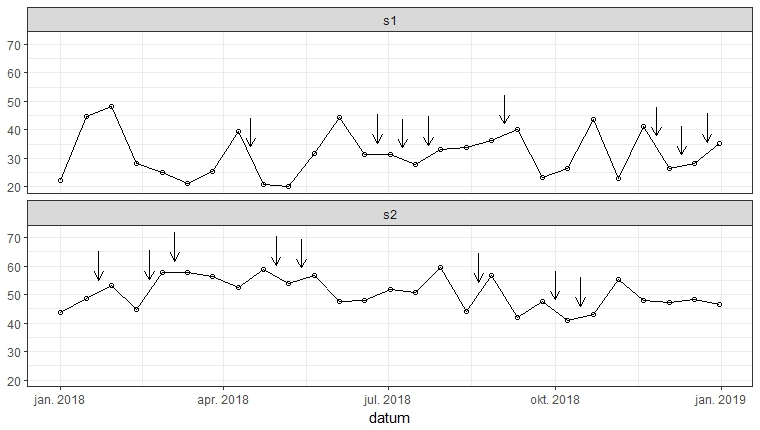# Point arrows to specific parts of the data

April 13, 2018
By

(This article was first published on Roman Luštrik not Inc. - R, and kindly contributed to R-bloggers)

tl;dr: Point arrows at your line at defined datum points.

Sometimes to really enhance your picture you need to point at some stuff. Here is a script which will plot vertical lines to show events in your datum plot.

```library(ggplot2)

# This script will draw points to parts of (interpolated) lines.

arrow.length <- 10
touchoff.distance <- 4 # distance between data and start of arrow
arrowhead.size <- 2.5 # in millimeters

# Simulate some data.
set.seed(12)
datum.seq <- seq(from = as.Date("2018-01-01"),
to = as.Date("2018-12-31"),
by = "2 week")

xy <- data.frame(datum = rep(datum.seq, times = 2))
xy\$count <- c(runif(length(datum.seq), min = 20, max = 50),
runif(length(datum.seq), min = 40, max = 60)
)
xy\$var1 <- rep(c("s1", "s2"), each = length(datum.seq))

# These are the points where we want to point to.
topoint <- data.frame(datum = sample(datum.seq, 16) - 7,
var1 = rep(c("s1", "s2"), each = 8))

# Function which interpolates per one variable.
interpolateOnDate <- function(xy, newdata) {
newdata <- newdata[newdata\$var1 == unique(xy\$var), ]
out <- approx(x = xy\$datum, y = xy\$count, xout = newdata\$datum)
newdata\$count <- out\$y
newdata
}

# Apply interpolation to each level of var1
res <- lapply(split(xy, f = xy\$var1),
FUN = interpolateOnDate,
newdata = topoint)

res <- do.call(rbind, res) # merge result

ggplot(xy, aes(x = datum, y = count)) +
theme_bw() + theme(axis.title.y = element_blank()) +
geom_point(shape = 1) +
geom_path() +
geom_segment(data = res, aes(x = datum, y = count + touchoff.distance,
xend = datum, yend = count + touchoff.distance + arrow.length),
arrow = arrow(length = unit(arrowhead.size, "mm"), ends = "first")) +
facet_wrap(~ var1, ncol = 1)
```R-bloggers.com offers daily e-mail updates about R news and tutorials on topics such as: Data science, Big Data, R jobs, visualization (ggplot2, Boxplots, maps, animation), programming (RStudio, Sweave, LaTeX, SQL, Eclipse, git, hadoop, Web Scraping) statistics (regression, PCA, time series, trading) and more...